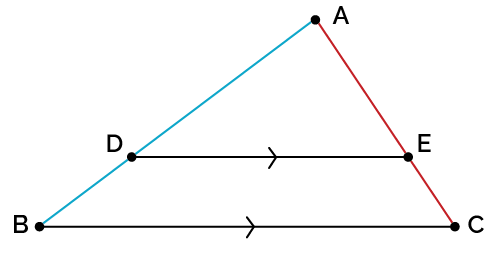# Triangle Proportionality Theorem Calculator

Created by Luis Fernando
Reviewed by Julia Żuławińska
Last updated: Jul 26, 2022

If you're studying geometry, a triangle proportionality theorem calculator like this one can be of great help.

Apart from knowing what is triangle proportionality theorem, you also need to know how to use it. With our tool, you'll learn how to find the length of the lines that make up the segments intersected by DE (look at the image).

If you want to dig deeper, in the , we present the triangle proportionality theorem proof.

## What is the triangle proportionality theorem?

In geometry, the triangle proportionality theorem says:

💡 If a line parallel to one side of a triangle intersects the other two sides, then the line divides those two sides proportionally.For example, for the triangle above in which the line DE (parallel to the BC side) intersects the other two sides (AB and AC) the triangle proportionality theorem says:

$\frac{\text{AB}}{\text{AD}} = \frac{\text{AC}}{\text{AE}}$

You can draw lines across the three directions, as long as they're parallel to one side, and the theorem will still be valid.

Now that you know the theory behind the calculation of the triangle proportionality theorem, you can look at other Omni tools similar to this triangle proportionality theorem calculator.

## FAQ

### What is a true statement about a 45-45-90 triangle?

A true statement about a 45-45-90 triangle is that the hypotenuse is √2 times as long as either leg. Let's explain why 👇

From the sine definition:

• sin(45°) = opposite/hypotenuse
• hypotenuse = (1/sin(45°)) × opposite
• hypotenuse = √2 × opposite

As the opposite sides are the legs, and both sides are equal:

hypotenuse = √2 × leg

### What is the converse of the triangle proportionality theorem?

As for any theorem, we can state a converse for the triangle proportionality theorem:

💡 If a line divides two sides of a triangle proportionally, that line must be parallel to the third side.

### How do I prove the triangle proportionality theorem?

To prove the triangle proportionality theorem, consider a triangle ABC with a line DE parallel to BC (image below)

1. As parallel lines form congruent angles, then:

∠ADE ≅ ∠ABC and ∠AED ≅ ∠ACB

2. From the AA similarity criteria:

△ABC ~ △ADE

3. As the triangles are proportional:
AB/AD = AC/AE

4. Using the denominator subtraction property:

(AB - AD)/AD = (AC - AE)/AE

5. As AB - AD = DB and AC - AE = EC, we can achieve the proof of the triangle proportionality theorem:

DB/AD = EC/AE

6. That's how you prove the triangle proportionality theorem!Luis FernandoInput three out of the four variables.
cm
AE
cm
DB
cm
EC
cm
People also viewed…

### Car heat

The hot car calculator shows you how fast the interior of a car heats up during a summers day.

### LCM

The LCM calculatorcalculates the least common multiple of two to fifteen numbers.

### Plant spacing

Planning out your garden? Try the plant spacing calculator.

### Unit rate

The unit rate calculator is here to give you your fraction's equivalent with one in the denominator (i.e. unit rate).